Back to Contents

# 3. Again and Again

In the previous chapter we used recursion to perform a calculation a variable number of times, and noted its limitations in Python. In this chapter, we learn the ordinary Python way of handling such situations.

### A fixed number of times

The `for` … `in` `range(`a, b`)` structure can be used to do something a number of times. For example, to print each of the numbers in the range in turn:The expressions inside the construct (or loop, as we call it) will be run once for each number in the range. Here is what we see on the screen:

``````0
1
2
3
4``````

We can see that the two arguments to the range function specify where to start, at 0, and where to stop, before 5. And so, the numbers 0 to 4 inclusive are printed. This behaviour is useful when programming, but unintuitive to humans. Let us write a function to print the numbers 1…n as a human might expect: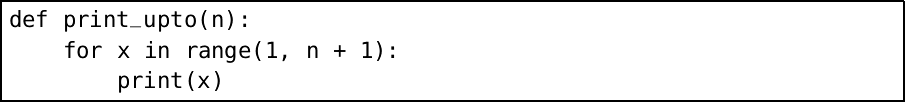So now, `print_upto(5)` will print this:

``````1
2
3
4
5``````

What if we want the numbers to be printed all on the same line? The Python `print` function moves to the next line by default. We can suppress this behaviour by supplying an alternative end to the line (`print` usually ends the line with what is called a newline character):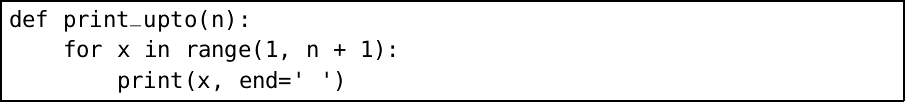Now the same statement `print_upto(5)` will print the numbers all on one line, with spaces in between:

``1 2 3 4 5``

We have used a second argument to the built-in `print` function – it is a named argument, with the name `end`. Such names help us remember which argument is which.

### One inside another

We can, of course, put one `for` loop inside another. Let us write a function to print a times table of any size: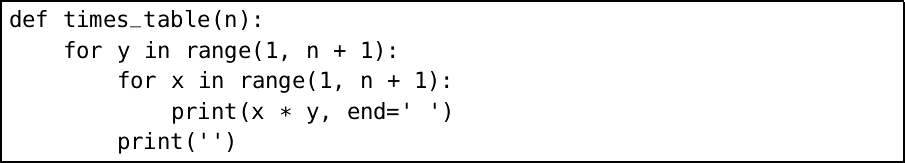We have used `print` with the empty string as its argument to move to a new line. Notice how the indentation helps to show the structure of the nested `for` structures. Here is the output of our new function for table size 5:

``````1 2 3 4 5
2 4 6 8 10
3 6 9 12 15
4 8 12 16 20
5 10 15 20 25``````

The columns are not lined up nicely, because some numbers need one digit to print and some need two. We can use the special letter `\t`, called a tab, to line the letters up (the `\` is called the escape character, and gives the letter following it special significance.)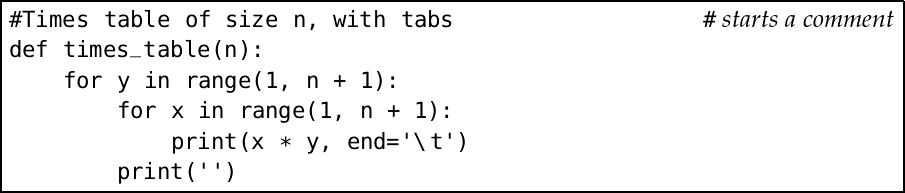Notice we have added a comment (beginning with `#`) to remind us that this is a different version of `times_table`. You can put as many comments as you like in your programs. Here is the output:

``````1       2       3       4       5
2       4       6       8       10
3       6       9       12      15
4       8       12      16      20
5       10      15      20      25``````

Tabs are a remnant of mechanical typewriter technology, where little metal stops could be placed in certain positions to line up columns at the touch of a button. We can do better by calculating the maximum width of any column, then printing enough spaces after each number: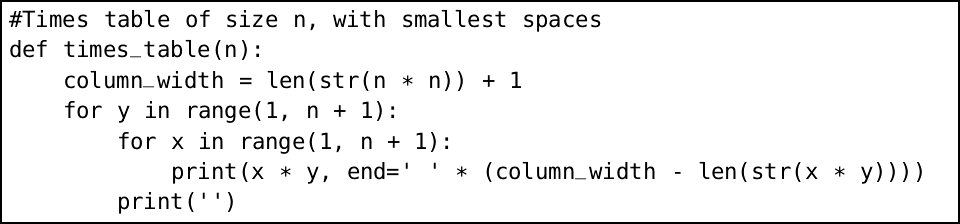The built-in function `str` converts a number to a string, and the built-in function `len` calculates the length of a string. We also use the `*` operator to build the string of many spaces from one space. For example, `’ ’ * 5` is `’      ’`. Here is the output:

``````1  2  3  4  5
2  4  6  8  10
3  6  9  12 15
4  8  12 16 20
5  10 15 20 25 ``````

Much better.

### Ranging over strings

We can also use to loop or iterate over things other than ranges of numbers. For example, if we use `for`… `in` with a string, each letter of the string will be selected in turn: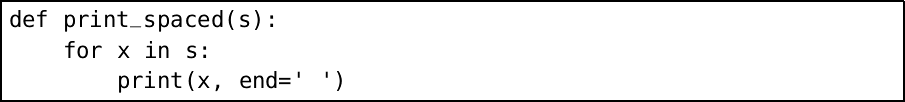The output of `print_spaced(’CHARLES’)` will be `C H A R L E S `.   In one of the questions you will be asked to find a way to remove that errant last space.

### An unknown number of times

What if we do not know how many times to repeat an action until we begin? We can use the `while` construct. For example, let us ask the user for a password before proceeding: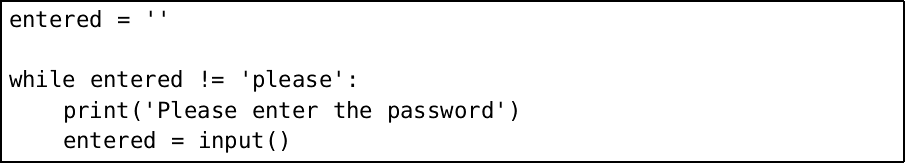The built-in `input` function, which has no arguments, allows the user to type in a line of text, returning when the Enter key is pressed. Here is a possible interaction:

``````Please enter the password
no

We cannot know how many times we might need to prompt the user for input, and so we could not have done this with a `for` loop. We can build our `while` loop into a function: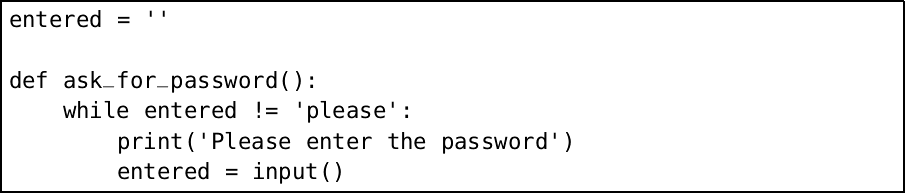Notice that our function also has no arguments, just like `input`, and returns nothing. However, it does not work:

``````Python
Traceback (most recent call last):
File "<stdin>", line 1, in <module>
UnboundLocalError: local variable 'entered' referenced
before assignment``````

### Local and global names

To write this function correctly, we must bring the definition of the `entered` variable inside the function definition – it will be a new, empty, `entered` each time the function is run: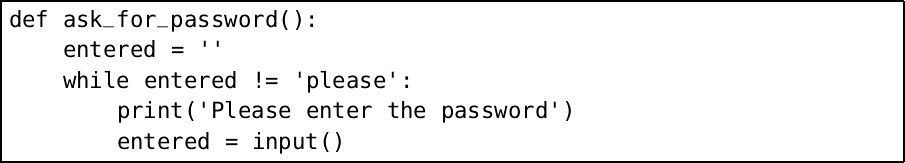This is called a local variable. In our first example, `entered` was a global variable. If we really wanted to use a global variable, we would write: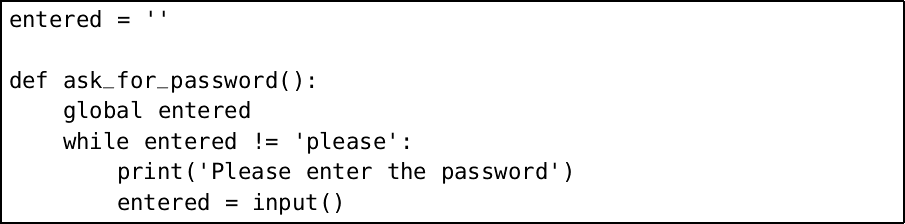There is a flaw in this program, however. If we run this version of the `ask_for_password` function twice then, on the second run, the variable `entered` will already have the correct password in it. So it is right that Python warns us of the dangers of global variables by requiring them to be explicitly declared. We will not use `global` in this book again.

## Common problems

It is important when building `for` loops to remember `range`, or we may be in for a nasty surprise:

``````Python
>>> for x in (0, 5):
...   print(x)
...
0
5``````

As we have already mentioned, it is important to remember that ranges begin at the first number given and stop before the second number given:

``````Python
>>> for x in range(1, 10):
...   print(x)
...
1
2
3
4
5
6
7
8
9``````

These are called half-open intervals. They are unintuitive to the beginner, but to the experienced programmer, they are more convenient, making sure that important properties hold. For example, that `len(range(a, b)) == a - b`.

When you begin to write programs with `input`, there is always the chance of problems with unexpected inputs. For example, expecting a number and using the built-in `int` function, which converts a string into a number:

``````Python
>>> a = input()
bob
>>> int(a)
Traceback (most recent call last):
File "<stdin>", line 1, in <module>
ValueError: invalid literal for int() with base 10: 'bob'``````

Later in the book, we will see how to deal cleanly with such situations.

## Summary

We have learned about two methods for repeating statements: `for` loops for a known number of times, and `while` loops when we do not know the number of times in advance. We have, along the way, converted from strings and numbers and back again with `str` and `int`, learned how to customize the `print` function, built bigger strings from smaller ones, and calculated the length of a string. We have started to build interactive programs, accepting input from the user.

We now have the tools to build a much wider and more interesting class of programs.

## Questions

1. The `range` construct can be given an extra, third, argument, the step. For example `range(0, 10, 2)` would iterate over 0, 2, 4, 6, and 8. Use this argument to write a function `print_down_from` which is the same as our `print_upto` function but prints the numbers in reverse order.

2. Our times table function, even in its final version, can put in too much space. This happens when only the last column contains the longest numbers, for example:

``````Python
>>> times_table(10)
1   2   3   4   5   6   7   8   9   10
2   4   6   8   10  12  14  16  18  20
3   6   9   12  15  18  21  24  27  30
4   8   12  16  20  24  28  32  36  40
5   10  15  20  25  30  35  40  45  50
6   12  18  24  30  36  42  48  54  60
7   14  21  28  35  42  49  56  63  70
8   16  24  32  40  48  56  64  72  80
9   18  27  36  45  54  63  72  81  90
10  20  30  40  50  60  70  80  90  100 ``````

Here only the final column has a number with three digits. Modify the function to correct this shortcoming.

3. Write a function `count_spaces` to count the number of spaces in a string.

4. Fix our `print_spaced` function to remove the errant final space. Hint: remember that the built-in function `len` can be used to find the length of a string.

5. Write a function which prints a sentence for the user to copy. Have the user type it in, and press Enter. Check if it is correct and print an appropriate message. If it is incorrect, keep going until it is correct.

6. Simplify our password example by supplying the prompt text directly as an argument to the `input` function. You will need to add the special string `’\n’`, called the newline character, to the end to move to the next line. Simplify it further by finding a way to remove the `entered` variable. You will need to use the `pass` keyword, which does nothing.

7. Use the `input` function to write an interactive guessing game. For example, we might see:

``````Python
>>>guessing_game()
Guess a number between 1 and 100
50
Lower!
15
Higher!
40
Lower!
35
Higher
37
Correct! You took 5 guesses.``````

You will need the built-in function `int` which converts a string to an integer. An arbitrary number between 1 and 100 may be obtained in the following way:

``````Python
>>> import random
>>> random.randint(1, 100)
44``````

(Note that we could also use `from` `random` `import` `randint` here and write `randint` instead of `random.randint`.)

8. Write a function to print a message in Morse code. Here is the table of codes: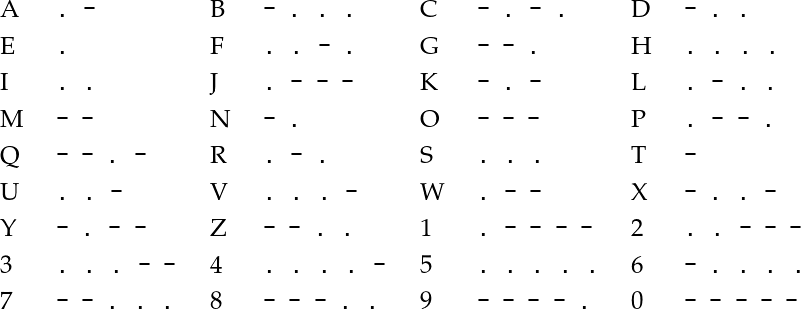There should be three spaces between letters, and seven spaces between words.